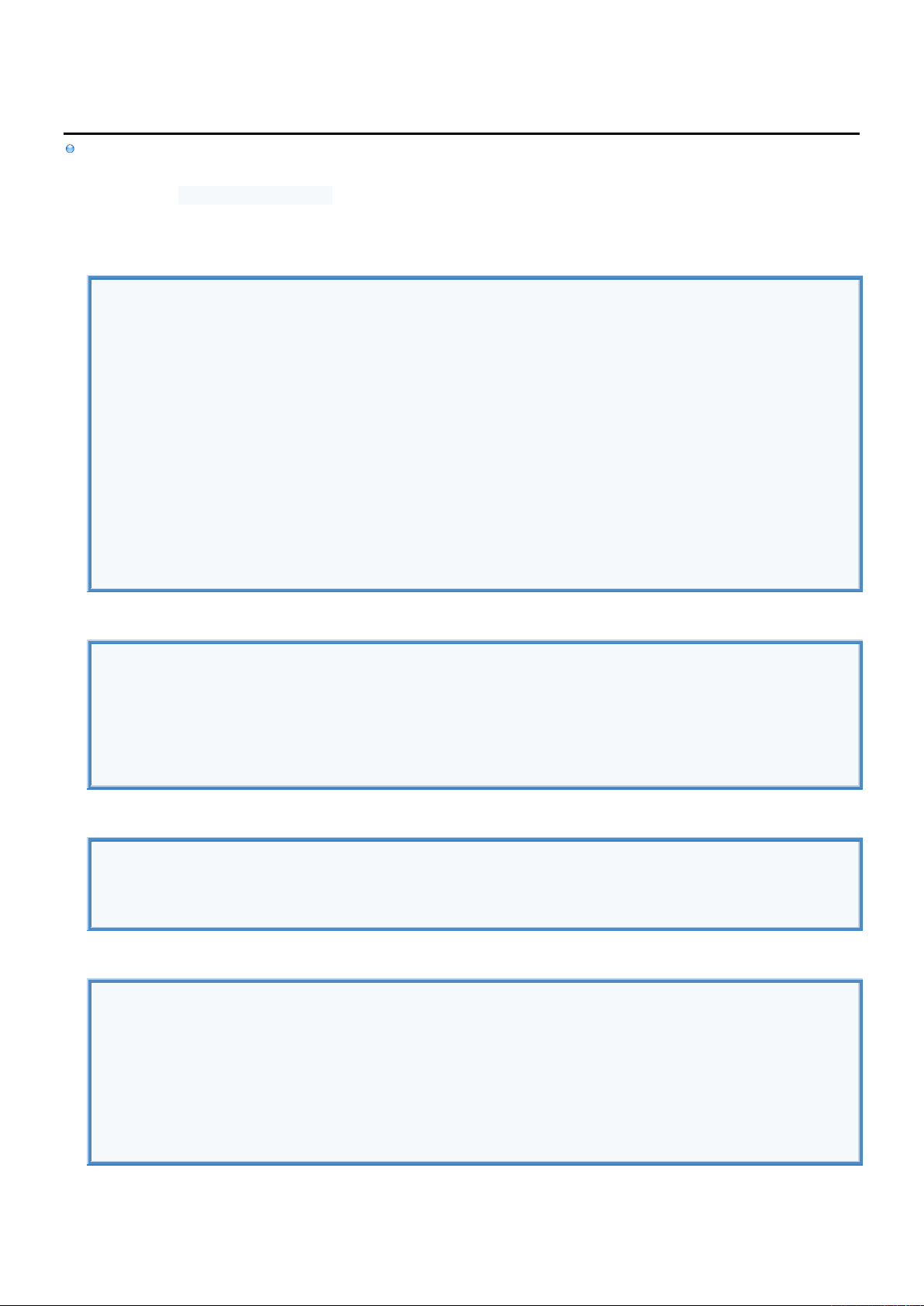2
2
.
.
6
6
.
.
2
2
S
S
t
t
r
r
i
i
n
n
g
g
I
I
n
n
f
f
o
o
[
[
R
R
]
]
String
is Class which contains sequence of characters
is the only Non-Primitive Data Type that can be instantiated using Literal (String Literal)
can also be instantiated using String Class
Declare String
//DECLARE USING STRING LITERAL.
String text = ""; //Empty string.text.length()=0,text.equals("")==true
text = "Hello World"; //Creates String Object with constant text "text".
text = "First line. \n Second line."; //Using escape character '\': \" \t \n \\
text = "Start" + "End"; //Connecting Strings.
//DECLARE USING STRING CONSTRUCTOR.
String text = null; //Calling any function on text throws Exception
text = new String(); //Empty string.
text = new String("text"); //Creates String Object from String Literal
text = new String(new byte[] {'J','o','h','n'}); //Creates String Object from byte array.
//DECLARE USING STRING METHODS.
String text = String.copyTextOf(new char[] {'J','o','h','n'}, 1, 2); //From index 1 take 2: "oh"
text = String.textOf (65 );
text = String.valueOf(65.0);
Modify String
String text = "012345 \n";
String modified = text.toUpperCase (); //Converts all characters to upper case.
modified = text.toLowerCase (); //Converts all characters to lower case.
modified = text.replace ('3', 'A' ); //Replace ALL occurrences of char '3' with 'A'.
modified = text.replaceFirst("23","AB"); //Replace FIRST occurrences of regex "23" with "AB".
modified = text.replaceAll ("23","AB"); //Replace ALL occurrences of regex "23" with "AB".
modified = text.replaceAll ("[\n\r]",""); //Remove all \n and \r characters.
Get Substring
String text = "012345";
String substr = text.substring (2); //Take substring from index 2 till the end. Result is "2345"
substr = text.substring (2,5); //Take substring from index 2 till 5-1=4. Result is "234".
substr = text.trim (); //Erases leading and trailing whitespaces: \t \n
Split String
String text = "zero<->one<->two<->three";
String[] tokens = text.split("<->" ); //Split string around "<->" regex. Gives tokens="one";
tokens = text.split("<->",3); //Create maximum 3 tokens. Gives tokens="two<->three";
tokens = text.split(""); //Split string around 1,3 and 5.
tokens = text.split("/" ); //Split string around '/'.
tokens = text.split("\\." ); //Split string around '.'.
tokens = text.split("\\\\" ); //Split string around '\'.
tokens = "" .split(";" ); //One token is returned resulting in tokens.length=1.
tokens = ";" .split(";" ); //No tokens are returned resulting in tokens.length=0.